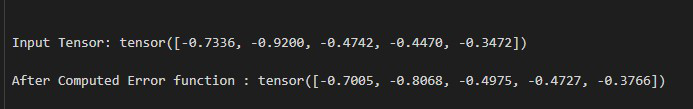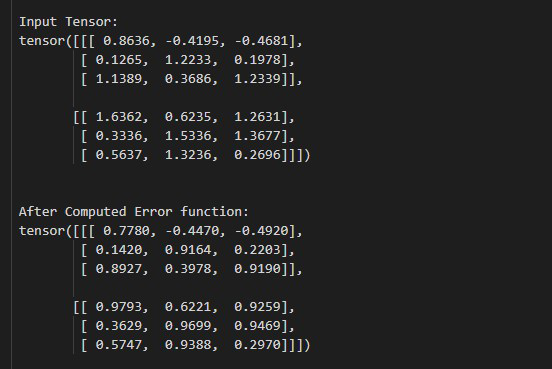# How to Compute the Error Function of a Tensor in PyTorch

• Last Updated : 03 May, 2022

In this article, we are going to cover how to compute the error function of a tensor in Python using PyTorch.

## torch.special.erf() method

We can compute the error function of a tensor by using torch.special.erf() method. This method accepts the input tensor of any dimension and it returns a tensor with a computed error function with the same dimension as the input tensor. The below syntax is used to compute the error function of a tensor.

Syntax: torch.special.erf(input)

Parameters:

• input: This is our input tensor.

Return: This method returns a tensor with computed error function of input tensor.

Example 1:

The following program is to understand how to compute the error function of the 1D tensor.

## Python3

 `# import required libraries``import` `torch` `# creating a 1D tensor``tens ``=` `torch.tensor([``-``0.7336``, ``-``0.9200``, ``-``0.4742``,``                     ``-``0.4470``, ``-``0.3472``])` `# print above created tensor``print``(``"\n Input Tensor:"``, tens)` `# compute the error function``er ``=` `torch.special.erf(tens)` `# Display result``print``(``"\n After Computed Error function :"``, er)`

Output:Example 2:

The following program is to know how to compute the error function of a batch of tensors.

## Python3

 `# import required libraries``import` `torch` `# creating a batch of tensor``tens ``=` `torch.tensor([[[``0.8636``, ``-``0.4195``, ``-``0.4681``],``                      ``[``0.1265``, ``1.2233``, ``0.1978``],``                      ``[``1.1389``, ``0.3686``, ``1.2339``]],``                     ``[[``1.6362``, ``0.6235``, ``1.2631``],``                      ``[``0.3336``, ``1.5336``, ``1.3677``],``                      ``[``0.5637``, ``1.3236``, ``0.2696``]]])` `# print above created tensor``print``(``"\n\n Input Tensor: \n"``, tens)` `# compute the error function``er ``=` `torch.special.erf(tens)` `# Display result``print``(``"\n\n After Computed Error function: \n"``, er)`

Output:My Personal Notes arrow_drop_up